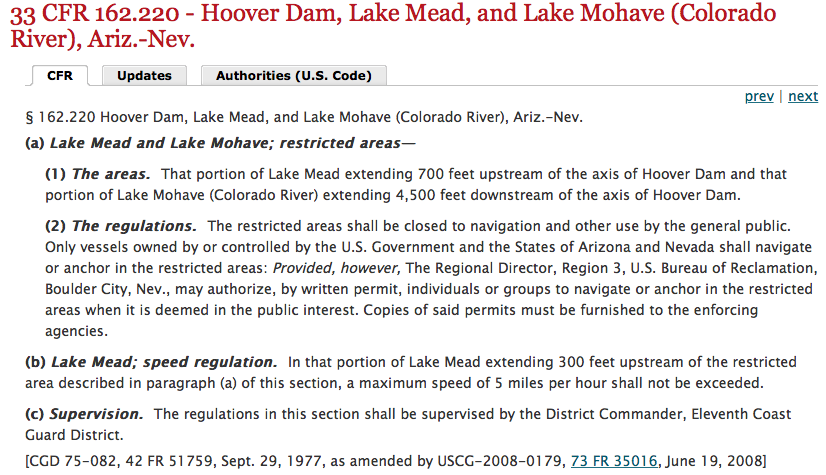## Monday, October 27, 2014

### 33 47 | Is CFR 33 the Writing on the Wall for Hoover Dam?Notice Dates:  9/29/1977 = 9+2+9+1+9+7+7 = 44; The World Trade Center's were opened April 4, or 4/4 6/19/2008 = 6+1+9+2+0+0+8 = 26

CFR stands for Code of Federal Regulations.
• Federal = 6+5+4+5+9+1+3 = 33
Notice how this Code of Federal Regulations pertains to the fact that only government higher-ups can get anywhere near the Hoover Dam.  In other words, if there is ever an act of terrorism there, we should all know who was responsible for it; the FEDERAL GOVERNMENT and the entities who hide behind it.

Also curious is the number of 62 and 222 within the CFR number sequence.
• Mason = 13+1+19+15+14 = 62
Let us also not overlook the name "Hoover Dam".
• Hoover Dam = 8+6+6+4+5+9+4+1+4 = 47
Recall it was Building 7 of 47-Floors that tumbled on 9/11.  That was blamed on "Osama bin Laden".
• Osama bin Laden = 6+1+1+4+1+2+9+5+3+1+4+5+5 = 47
Again, the writing is on the wall... of the largest dam in the United States.  The biggest teller is the date the document was originally published, producing a Gematria of "44".
• 9/29/1977 = 9+2+9+1+9+7+7 = 44
• 9/29/77 = 9+29+77 = 115
The World Trade Center Complex opened April 4, 1973, five years to the day of the MLK Jr. assassination.  Notice the date of 4/4.  The Gematria of the word "kill" is "44".
• Kill = 11+9+12+12 = 44
• Also notice how the date 9/29 is much like 9/11 in numerology because 2+9 = 11
• 9/29 = 9/11 in numerology...

1.Man, Look at the two dates on the bottom. Sept./29/1977.. 9+2+9+1+9+7+7= 44. and June/19/2008.. 6+1+9+2+0+0+8= 26. Also, looking at the 220 in the 162.220 I think of the number of floors combined of the two towers.. 110+110. Have you found much of 220 showing up in your research or 162 for that matter?

1.I think 220 is much the same as 22, but yes with the sum of the Twin Towers... As for 162, I think to them it is the same as 62, which is what the word Mason sums to in Gematria.

I did the numerology for both of those dates and then forgot to include them in the post, lol. Thanks for the reminder.

2.Genesis (book 1) chapter (6) up to verse (22) is about Noah and the flood; v.2?

1.Very good connection.

3.Zach check this out...... http://endtimesforecaster.blogspot.com/2014/10/the-next-possible-friday-crescent-moon.html?utm_source=BP_recent

1.Excellent find, 322... like the caliber of the AR-15 they so often code into the same stories, and of course, like Skull and Bones, which is a 44-minute drive to Sandy Hook from their campus doors.

4.What is up with the number 115 showing up so much lately? April 25, 2015 (115th day of the year) Nepal / Himalayan earthquake. Maybe 'September seventh' = 196 (250th day of the year with 115 days remaining) is something to keep an eye on.